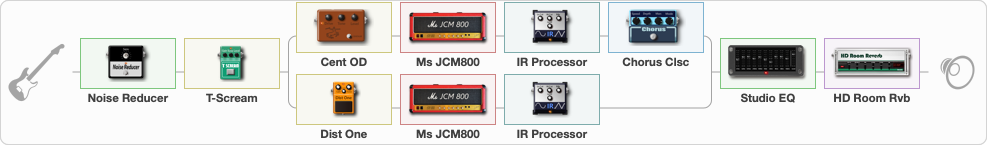# JH KILL EM ALL

Discussion in 'ToneLib-GFX presets' started by grigoriy0192873, Mar 20, 2023.

1. ### grigoriy0192873Active Member

JH KILL EM ALL

Preset name: JH KILL EM ALL

Effects chain:Effect: "Noise Reducer" (Dynamics / Filter), active - "yes"
{
"Sens" = 65
"Mode" = Hard
}

Effect: "T-Scream" (Overdrive / Distortion), active - "yes"
{
"Drive" = 75
"Tone" = 40
"Level" = 35
}

Effect: "Splitter" (Dynamics / Filter)
{
"A-Bypass" = Off
"A-Pan" = 0
"A-Level" = 55
"B-Bypass" = Off
"B-Pan" = 0
"B-Level" = 55
"Width" = 0

'A' branch:
{

Effect: "Cent OD" (Overdrive / Distortion), active - "yes"
{
"Drive" = 55
"Tone" = 45
"Level" = 45
}

Effect: "Ms JCM800" (Amp simulators), active - "yes"
{
"Gain" = 85
"Bass" = 55
"Middle" = 50
"Treble" = 65
"Presence" = 45
"Master" = 55
"Level (dB)" = 3
}

Effect: "IR Processor" (Cabinets), active - "yes"
{
"IR" = killem_IR
"Low Cut (Hz)" = 125
"Hi Cut (kHz)" = 6.5
"Mix" = 100
"Level (dB)" = -4
}

Effect: "Chorus Clsc" (Modulation / Sfx), active - "yes"
{
"Speed" = 1.5
"Depth" = 25
"Center" = 5.5
"Mode" = Stereo
}
}
'B' branch:
{

Effect: "Dist One" (Overdrive / Distortion), active - "yes"
{
"Drive" = 65
"Tone" = 45
"Level" = 45
}

Effect: "Ms JCM800" (Amp simulators), active - "yes"
{
"Gain" = 85
"Bass" = 55
"Middle" = 50
"Treble" = 65
"Presence" = 45
"Master" = 55
"Level (dB)" = 3
}

Effect: "IR Processor" (Cabinets), active - "yes"
{
"IR" = killem_IR
"Low Cut (Hz)" = 125
"Hi Cut (kHz)" = 6.5
"Mix" = 100
"Level (dB)" = -4
}
}
}

Effect: "Studio EQ" (Dynamics / Filter), active - "yes"
{
"31 Hz" = -6
"62 Hz" = -1
"125 Hz" = 3
"250 Hz" = 3
"500 Hz" = -3
"1 kHz" = -4
"2 kHz" = 0
"4 kHz" = 3
"8 kHz" = 3
"16 kHz" = -15
"Level (dB)" = 5
}

Effect: "HD Room Rvb" (Reverberation), active - "yes"
{
"Time" = 2.5
"PreLPF" = 0
"PreDelay" = 0
"HiDamp" = 100
"LoGain" = -12.0
"Mix" = 25
}

Note: You will need to download and install the ToneLib-GFX software to use the preset.

File size:
4.6 KB
Views:
1,597